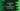# JavaScript program to find the nearest number in an array## Nearest number in an array :

Our problem is to find the nearest number in an array specific to a given number. For example, if our array is [1,5,10,15,20] and if the given number is 18, the output of the program should be 20 as this is the nearest number to 18 in this array. Again, if the number is 16, it will print 15. Note that we are checking in both ways to find the nearest number.

I will show you different ways to solve this. For each program, the array and the number is already given. The JavaScript program will find the nearest member and print that out. The main part is written in a separate function for each case.

## Method 1 : Using a loop :

• This is a straight forward way to find out the nearest number. In simple words :

Use one variable to store the current closest number. Assign its value to the first element of the array. We will keep updating it and it will hold the final result value at the end of the program.

• Run one for loop to iterate through the numbers one by one.

• For each number, find its difference to the given number. Find if it is less than the difference of the given number and current closest or not. If yes, assign it as the closest.

• Keep updating the closest variable with the loop and return it at the end of the program.

Below is the complete JavaScript program :

``````const findClosest = (arr,num) => {
if(arr == null) {
return
}

let closest = arr;
for(let item of arr){
if(Math.abs(item - num)<Math.abs(closest - num)){
closest = item;
}
}
return closest;
}

const array = [10,20,30,40,50,60,70,80,90];
const num = 36;
console.log(findClosest(array,num));
``````

### Explanation :

• Here, findClosest function is used to find the closest number in an array with respect to a given number. It takes two parameters : arr is the array with all numbers and num is the given number.

• If the provided array is null, return.

• closest variable will hold the nearest value to num. Initialize it with the first element of the array.

• Run one for-of loop to iterate through the elements of the array.

• For each element, check the absolute difference of current element, given number and current closest, given number. Update the value of closest based on this comparison.

• Finally, return the closest number found.

## Method 2 : Using reduce :

reduce function calculates one result from a JavaScript array. It takes one function and use it to find the final result. We can use reduce to find out the closest element to a given value in an array. Below is the complete program :

``````const findClosest = (arr,num) => {
if(arr == null){
return
}

return arr.reduce((prev,current) => Math.abs(current - num)<Math.abs(prev - num) ? current : prev);
}

const array = [10,20,30,40,50,60,70,80,90];
const num = 36;
console.log(findClosest(array,num));
``````

### Explanation :

We are using same function name findClosest as the previous example. It take one array and the number as arguments and returns the closest member found relative to the given number.

• The main concept of this solution is same as the previous example. It calculates the absolute difference of the previous and current number with respect to the given number and returns the closest value.

• At the end, it results the closest number with respect to the given number.

## Method 3: Using sort :

In JavaScript, sorting can be done in many ways. Not only increasing and decreasing. You can define the rule how you want to sort. We can write our program to sort the array such that the number that is closest to the given number moves to the end of the array as like below :

``````const findClosest = (arr,num) => {
if(array == null){
return
}
return arr.sort((a,b) => Math.abs(b - num) - Math.abs(a-num)).pop();
}

const array = [10,20,30,40,50,60,70,80,90];
const num = 36;
console.log(findClosest(array,num));
``````

### Explanation :

Here, we are sorting the array in such a way that the number that is closest to the given number moves to the end of the array. The final array after sorting in the above example will be [ 90, 80, 70, 10, 60, 20, 50, 30, 40 ]. Next, we are using pop() to remove the last element.